LM158QML
LM158QML Low Power Dual Operational Amplifiers
Literature Number: SNOSAP3E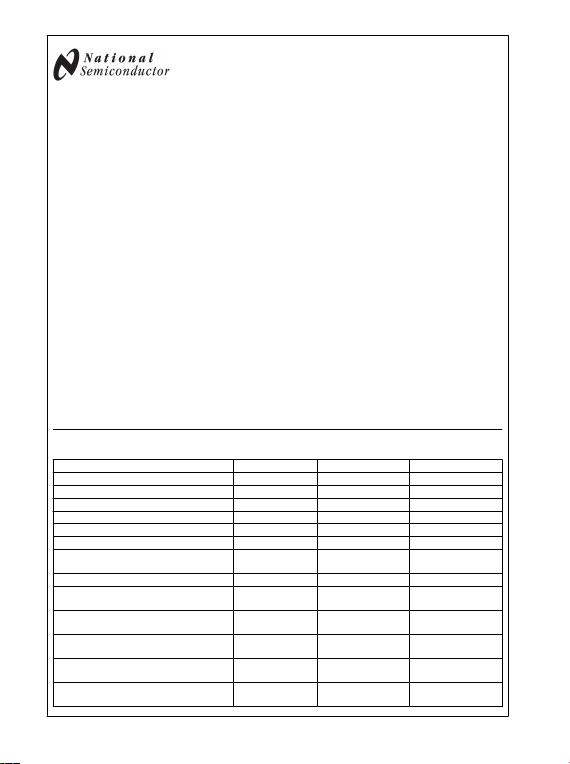January 13, 2009
LM158QML
Low Power Dual Operational Amplifiers
General Description
The LM158 series consists of two independent, high gain, in-
ternally frequency compensated operational amplifiers which
were designed specifically to operate from a single power
supply over a wide range of voltages. Operation from split
power supplies is also possible and the low power supply
current drain is independent of the magnitude of the power
supply voltage.
Application areas include transducer amplifiers, dc gain
blocks and all the conventional op amp circuits which now can
be more easily implemented in single power supply systems.
For example, the LM158 series can be directly operated off
of the standard +5V power supply voltage which is used in
digital systems and will easily provide the required interface
electronics without requiring the additional ±15V power sup-
plies.
Unique Characteristics
In the linear mode the input common-mode voltage range
includes ground and the output voltage can also swing to
ground, even though operated from only a single power
supply voltage.
The unity gain cross frequency is temperature
compensated.
The input bias current is also temperature compensated.
Two internally compensated op amps
Eliminates need for dual supplies
Allows direct sensing near Gnd and VO also goes to Gnd
Compatible with all forms of logic
Power drain suitable for battery operation
Features
Available with radiation guarantee
High Dose Rate 100 krad(Si)
ELDRS Free 100 krad(Si)
Internally frequency compensated for unity gain
Large dc voltage gain: 100 dB
Wide bandwidth (unity gain): 1 MHz
(temperature compensated)
Wide power supply range:
Single supply: 3V to 32V
or dual supplies: ±1.5V to ±16V
Very low supply current drain (500 μA) − essentially
independent of supply voltage
Low input offset voltage: 2 mV
Input common-mode voltage range includes ground
Differential input voltage range equal to the power supply
voltage
Large output voltage swing: 0V to V+ − 1.5V
Ordering Information
NS Part Number SMD Part Number NS Package Number Package Description
LM158H/883 H08C 8LD T0–99 Metal Can
LM158J/883 5962–8771001PA J08A 8LD Ceramic DIP
LM158H-SMD 5962–8771001GA H08C 8LD T0–99 Metal Can
LM158AH/883 5962–8771002GA H08C 8LD T0–99 Metal Can
LM158AJ/883 5962–8771002PA J08A 8LD Ceramic DIP
LM158AWG/883 5962–8771002QXA WG10A 10LD Ceramic SOIC
LM158AHRQMLV
HIGH DOSE RATE ONLY (Note 11)
5962R8771002VGA
H08C 8LD T0–99 Metal Can
LM158AJ-QMLV 5962–8771002VPA J08A 8LD Ceramic DIP
LM158AJRQMLV
HIGH DOSE RATE ONLY (Note 11)
5962R8771002VPA
J08A 8LD Ceramic DIP
LM158AWGRQMLV
HIGH DOSE RATE ONLY (Note 11)
5962R8771002VXA
WG10A 10LD Ceramic SOIC
LM158A MDR
HIGH DOSE RATE ONLY DIE (Notes 1, 11)
5962R8771002V9A
LM158AHRLQMLV
ELDRS FREE ONLY (Note 12)
5962R8771003VGA
H08C 8LD T0–99 Metal Can
LM158AJRLQMLV
ELDRS FREE ONLY (Note 12)
5962R8771003VPA
J08A 8LD Ceramic DIP
© 2009 National Semiconductor Corporation 201502 www.national.com
LM158QML Low Power Dual Operational Amplifiers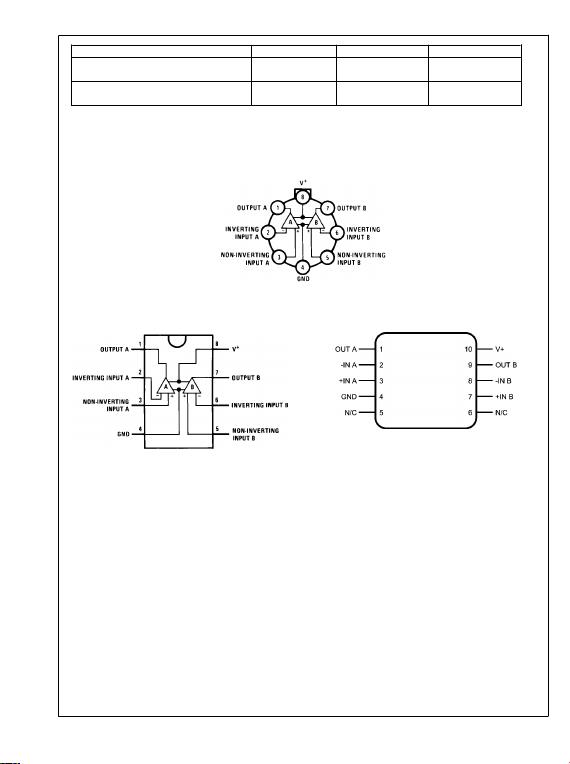NS Part Number SMD Part Number NS Package Number Package Description
LM158AWGRLQMLV
ELDRS FREE ONLY (Note 12)
5962R8771003VXA
WG10A 10LD Ceramic SOIC
LM158A MDE
ELDRS FREE ONLY DIE (Notes 1, 12)
5962R8771003V9A
Note 1: FOR ADDITIONAL DIE INFORMATION, PLEASE VISIT THE HI REL WEB SITE AT: www.national.com/analog/space/level_die
Connection Diagrams
Metal Can Package
20150201
Top View
See NS Package Number H08C
DIP Package
20150204
Top View
See NS Package Number J08A
10LD Ceramic SOIC
20150202
Top View
See NS Package Number WG10A
www.national.com 2
LM158QML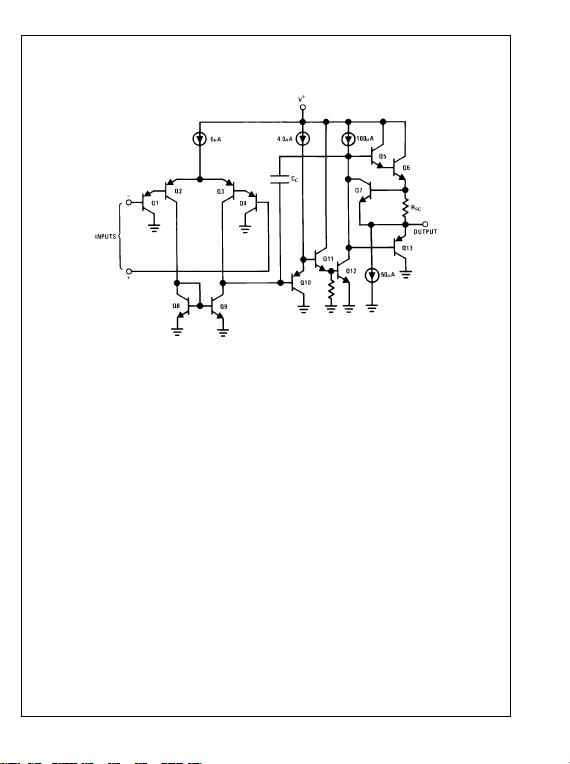Schematic Diagram
(Each Amplifier)
20150203
3 www.national.com
LM158QML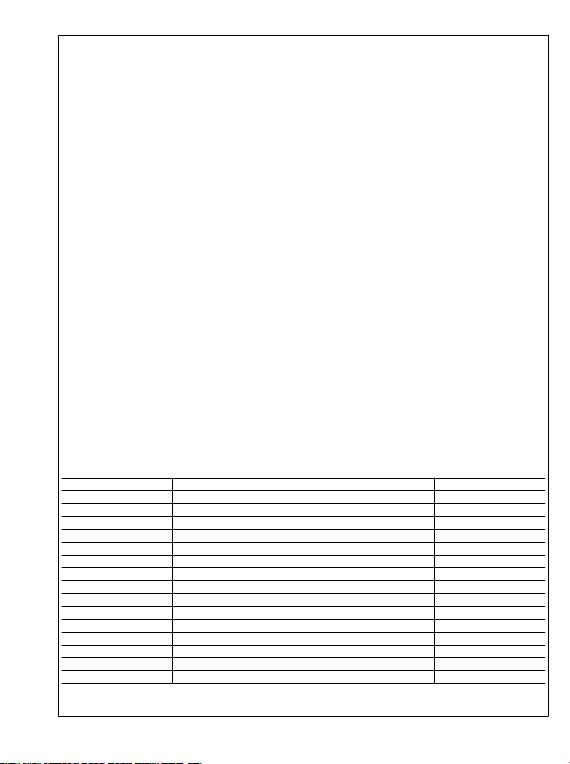Absolute Maximum Ratings (Note 2)
Supply Voltage, V+32VDC
Differential Input Voltage 32VDC
Input Voltage −0.3VDC to +32VDC
Power Dissipation (Note 3) 830 mW
Output Short-Circuit to GND(Note 4)
(One Amplifier)
V+ 15VDC and TA = 25°C
Continuous
Maximum Junction Temperature (TJmax) 150°C
Input Current (VI < −0.3V)(Note 5) 50 mA
Operating Temperature Range −55°C TA +125°C
Storage Temperature Range −65°C TA +150°C
Lead Temperature (Soldering, 10 seconds)
Metal Can 300°C
Ceramic DIP 260°C
Ceramic SOIC 260°C
Thermal Resistance
θJA
Metal Can (Still Air) 155°C/W
Metal Can (500LF/Min Air Flow) 80°C/W
Ceramic DIP (Still Air) 132°C/W
Ceramic DIP (500LF/Min Air Flow) 81°C/W
Ceramic SOIC (Still Air) 195°C/W
Ceramic SOIC (500LF/Min Air Flow) 131°C/W
θJC
Metal Can 42°C/W
Ceramic DIP 23°C/W
Ceramic SOIC 33°C/W
Package Weight
Metal Can 1,000mg
Ceramic DIP 1,100mg
Ceramic SOIC 220mg
ESD Tolerance (Note 8) 250V
Quality Conformance Inspection
Mil-Std-883, Method 5005 - Group A
Subgroup Description Temp °C
1 Static tests at +25
2 Static tests at +125
3 Static tests at -55
4 Dynamic tests at +25
5 Dynamic tests at +125
6 Dynamic tests at -55
7 Functional tests at +25
8A Functional tests at +125
8B Functional tests at -55
9 Switching tests at +25
10 Switching tests at +125
11 Switching tests at -55
12 Settling time at +25
13 Settling time at +125
14 Settling time at -55
www.national.com 4
LM158QML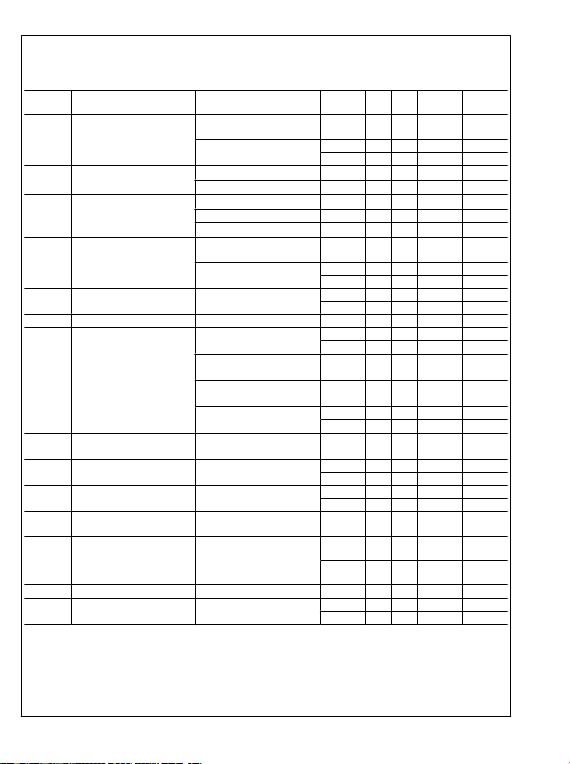LM158 Electrical Characteristics SMD 5962–8771001
DC Parameters
The following conditions apply, unless otherwise specified. All voltages referenced to device ground.
Symbol Parameter Conditions Notes Min Max Units Sub-
groups
ICC Power Supply Current +VCC = 5V, RL = 100K,
VO = 1.4V
1.2 mA 1, 2, 3
+VCC = 30V, RL = 100K,
VO = 1.4V
3.0 mA 1
4.0 mA 2, 3
VOH Output Voltage High +VCC = 30V, RL = 2K 26 V 1, 2, 3
+VCC = 30V, RL = 10K 27 V 1, 2, 3
VOL Output Voltage Low +VCC = 30V, RL = 10K 20 mV 1, 2, 3
+VCC = 30V, ISink = 1µA 20 mV 1, 2, 3
+VCC = 5V, RL = 10K 20 mV 1, 2, 3
ISink Output Sink Current +VCC = 15V, VO = 200mV,
+VI = 0V, -VI = +65mV
12 µA 1
+VCC = 15V, VO = 2V,
+VI = 0V, -VI = +65mV
10 mA 1
5.0 mA 2, 3
ISource Output Source Current +VCC = 15V, VO = 2V,
+VI = 0V, -VI = -65mV
-20 mA 1
-10 mA 2, 3
IOS Short Circuit Current +VCC = 5V, VO = 0V -60 mA 1
VIO Input Offset Voltage +VCC = 30V, VCM = 0V,
RS = 50Ω, VO = 1.4V
-5.0 5.0 mV 1
-7.0 7.0 mV 2, 3
+VCC = 30V, VCM = 28.5V,
RS = 50Ω, VO = 1.4V
-5.0 5.0 mV 1
+VCC = 30V, VCM = 28V,
RS = 50Ω, VO = 1.4V
-7.0 7.0 mV 2, 3
+VCC = 5V, VCM = 0V,
RS = 50Ω, VO = 1.4V
-5.0 5.0 mV 1
-7.0 7.0 mV 2, 3
CMRR Common Mode Rejection Ratio +VCC = 30V, RS = 50Ω
VI = 0V to 28.5V,
70 dB 1
±IIB Input BIas Current +VCC = 5V, VCM = 0V (Note 6) -150 -1.0 nA 1
(Note 6) -300 -1.0 nA 2, 3
IIO Input Offset Current +VCC = 5V, VCM = 0V -30 30 nA 1
-100 100 nA 2, 3
PSRR Power Supply Rejection Ratio +VCC = 5V to 30V,
VCM = 0V
65 dB 1
VCM Common Mode Voltage Range +VCC = 30V (Note 7),
(Note 9)
28.5 V 1
(Note 7),
(Note 9)
28.0 V 2, 3
VDiff Differential Input Voltage (Note 10) 32 V 1, 2, 3
AVS Large Signal Gain +VCC = 15V, RL = 2KΩ,
VO = 1V to 11V
50 V/mV 4
25 V/mV 5, 6
5 www.national.com
LM158QML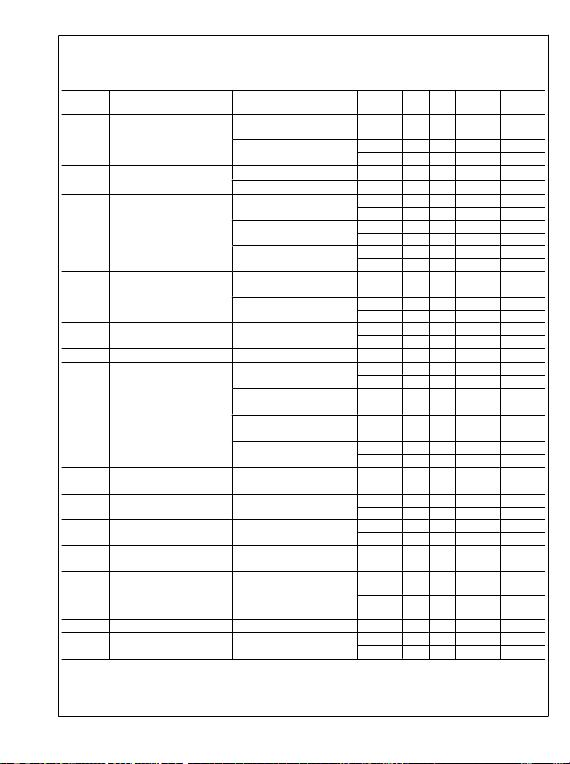LM158A Electrical Characteristics SMD 5962–8771002, High Dose Rate
DC Parameters
The following conditions apply, unless otherwise specified. All voltages referenced to device ground.
Symbol Parameter Conditions Notes Min Max Units Sub-
groups
ICC Power Supply Current +VCC = 5V, RL = 100K,
VO = 1.4V
1.2 mA 1, 2, 3
+VCC = 30V, RL = 100K,
VO = 1.4V
3.0 mA 1
4.0 mA 2, 3
VOH Output Voltage High +VCC = 30V, RL = 2K 26 V 1, 2, 3
+VCC = 30V, RL = 10K 27 V 1, 2, 3
VOL Output Voltage Low +VCC = 30V, RL = 10K 40 mV 1
100 mV 2, 3
+VCC = 30V, ISink = 1µA 40 mV 1
100 mV 2, 3
+VCC = 5V, RL = 10K 40 mV 1
100 mV 2, 3
ISink Output Sink Current +VCC = 15V, VO = 200mV,
+VI = 0V, -VI = +65mV
12 µA 1
+VCC = 15V, VO = 2V,
+VI = 0V, -VI = +65mV
10 mA 1
5.0 mA 2, 3
ISource Output Source Current +VCC = 15V, VO = 2V,
+VI = 0V, -VI = -65mV
-20 mA 1
-10 mA 2, 3
IOS Short Circuit Current +VCC = 5V, VO = 0V -60 mA 1
VIO Input Offset Voltage +VCC = 30V, VCM = 0V,
RS = 50Ω, VO = 1.4V
-2.0 2.0 mV 1
-4.0 4.0 mV 2, 3
+VCC = 30V, VCM = 28.5V,
RS = 50Ω, VO = 1.4V
-2.0 2.0 mV 1
+VCC = 30V, VCM = 28V,
RS = 50Ω, VO = 1.4V
-4.0 4.0 mV 2, 3
+VCC = 5V, VCM = 0V,
RS = 50Ω, VO = 1.4V
-2.0 2.0 mV 1
-4.0 4.0 mV 2, 3
CMRR Common Mode Rejection Ratio +VCC = 30V, RS = 50Ω
VI = 0V to 28.5V,
70 dB 1
±IIB Input BIas Current +VCC = 5V, VCM = 0V (Note 6) -50 -1.0 nA 1
(Note 6) -100 -1.0 nA 2, 3
IIO Input Offset Current +VCC = 5V, VCM = 0V -10 10 nA 1
-30 30 nA 2, 3
PSRR Power Supply Rejection Ratio +VCC = 5V to 30V,
VCM = 0V
65 dB 1
VCM Common Mode Voltage Range +VCC = 30V (Notes 7,
9)
28.5 V 1
(Notes 7,
9)
28.0 V 2, 3
VDiff Differential Input Voltage (Note 10) 32 V 1, 2, 3
AVS Large Signal Gain +VCC = 15V, RL = 2KΩ,
VO = 1V to 11V
50 V/mV 4
25 V/mV 5, 6
www.national.com 6
LM158QML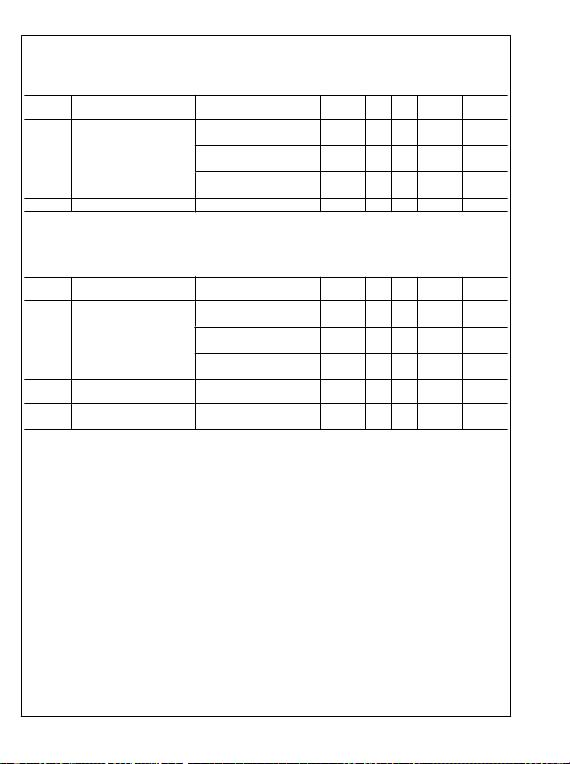SMD 5962–8771002, High Dose Rate
DC Drift Parameters
The following conditions apply, unless otherwise specified. All voltages referenced to device ground.
Delta calculations are performed on QMLV devices at Group B, Subgroup 5 only.
Symbol Parameter Conditions Notes Min Max Units Sub-
groups
VIO Input Offset Voltage +VCC = 30V, VCM = 0V,
RS = 50Ω, VO = 1.4V
-0.5 0.5 mV 1
+VCC = 30V, VCM = 28.5V,
RS = 50Ω, VO = 1.4V
-0.5 0.5 mV 1
+VCC = 5V, VCM = 0V,
RS = 50Ω, VO = 1.4V
-0.5 0.5 mV 1
±IIB Input Bias Current +VCC = 5V, VCM = 0V (Note 6) -10 10 nA 1
SMD 5962–8771002, High Dose Rate
100K Post Radiation Limits @ +25°C (Note 11)
DC Parameters
The following conditions apply, unless otherwise specified. All voltages referenced to device ground.
Symbol Parameter Conditions Notes Min Max Units Sub -
groups
VIO Input Offset Voltage +VCC = 30V, VCM = 0V,
RS = 50Ω, VO = 1.4V
(Note 11) -4.0 4.0 mV 1
+VCC = 30V, VCM = 28.5V,
RS = 50Ω, VO = 1.4V
(Note 11) -4.0 4.0 mV 1
+VCC = 5V, VCM = 0V,
RS = 50Ω, VO = 1.4V
(Note 11) -4.0 4.0 mV 1
±IIB Input Bias Current +VCC = 5V, VCM = 0V (Notes 6,
11)
-60 -1.0 nA 1
ICC Power Supply Current +VCC = 5V, RL = 100K,
VO = 1.4V
(Note 11) 1.5 mA 1
7 www.national.com
LM158QML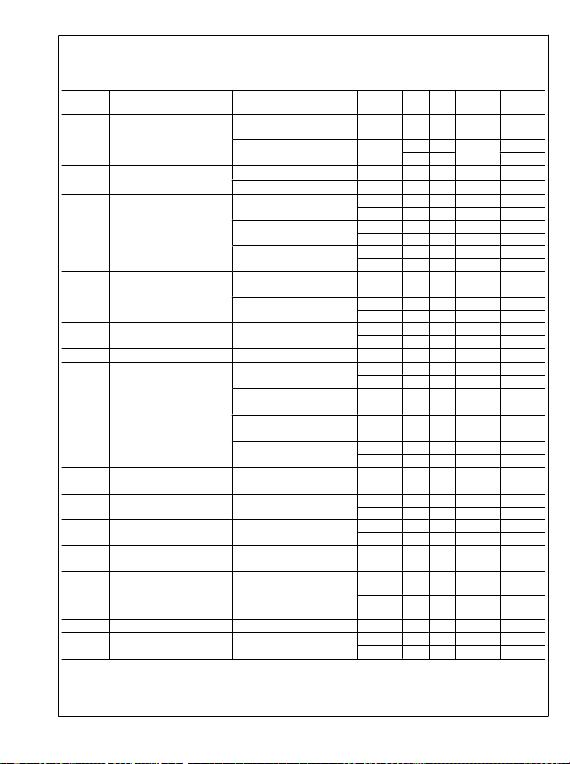LM158A Electrical Characteristics SMD 5962–8771003 ELDRS Free Only
DC Parameters
The following conditions apply, unless otherwise specified. All voltages referenced to device ground.
Symbol Parameter Conditions Notes Min Max Units Sub-
groups
ICC Power Supply Current +VCC = 5V, RL = 100K,
VO = 1.4V
1.2 mA 1, 2, 3
+VCC = 30V, RL = 100K,
VO = 1.4V
3.0 mA 1,
4.0 2, 3
VOH Output Voltage High +VCC = 30V, RL = 2K 26 V 1, 2, 3
+VCC = 30V, RL = 10K 27 V 1, 2, 3
VOL Output Voltage Low +VCC = 30V, RL = 10K 40 mV 1
100 mV 2, 3
+VCC = 30V, ISink = 1µA 40 mV 1
100 mV 2, 3
+VCC = 5V, RL = 10K 40 mV 1
100 mV 2, 3
ISink Output Sink Current +VCC = 15V, VO = 200mV,
+VI = 0V, -VI = +65mV
12 µA 1
+VCC = 15V, VO = 2V,
+VI = 0V, -VI = +65mV
10 mA 1
5.0 mA 2, 3
ISource Output Source Current +VCC = 15V, VO = 2V,
+VI = 0V, -VI = -65mV
-20 mA 1
-10 mA 2, 3
IOS Short Circuit Current +VCC = 5V, VO = 0V -60 mA 1
VIO Input Offset Voltage +VCC = 30V, VCM = 0V,
RS = 50Ω, VO = 1.4V
-2.0 2.0 mV 1
-4.0 4.0 mV 2, 3
+VCC = 30V, VCM = 28.5V,
RS = 50Ω, VO = 1.4V
-2.0 2.0 mV 1
+VCC = 30V, VCM = 28V,
RS = 50Ω, VO = 1.4V
-4.0 4.0 mV 2, 3
+VCC = 5V, VCM = 0V,
RS = 50Ω, VO = 1.4V
-2.0 2.0 mV 1
-4.0 4.0 mV 2, 3
CMRR Common Mode Rejection Ratio +VCC = 30V, RS = 50Ω
VI = 0V to 28.5V,
70 dB 1
±IIB Input BIas Current +VCC = 5V, VCM = 0V (Note 6) -50 -1.0 nA 1
(Note 6) -100 -1.0 nA 2, 3
IIO Input Offset Current +VCC = 5V, VCM = 0V -10 10 nA 1
-30 30 nA 2, 3
PSRR Power Supply Rejection Ratio +VCC = 5V to 30V,
VCM = 0V
65 dB 1
VCM Common Mode Voltage Range +VCC = 30V (Note 7),
(Note 9)
28.5 V 1
(Note 7),
(Note 9)
28.0 V 2, 3
VDiff Differential Input Voltage (Note 10) 32 V 1, 2, 3
AVS Large Signal Gain +VCC = 15V, RL = 2KΩ,
VO = 1V to 11V
50 V/mV 4
25 V/mV 5, 6
www.national.com 8
LM158QML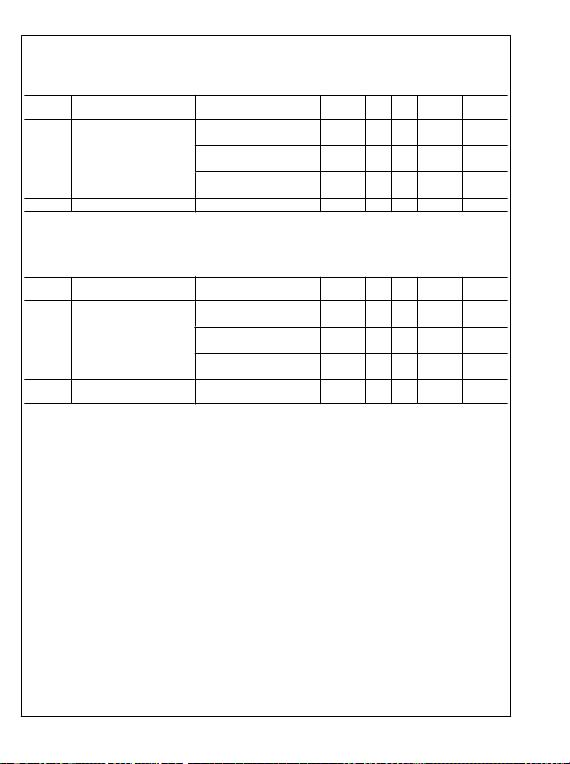SMD 5962–8771003, ELDRS Free Only
DC Drift Parameters
The following conditions apply, unless otherwise specified. All voltages referenced to device ground.
Delta calculations are performed on QMLV devices at Group B, Subgroup 5 only.
Symbol Parameter Conditions Notes Min Max Units Sub-
groups
VIO Input Offset Voltage +VCC = 30V, VCM = 0V,
RS = 50Ω, VO = 1.4V
-0.5 0.5 mV 1
+VCC = 30V, VCM = 28.5V,
RS = 50Ω, VO = 1.4V
-0.5 0.5 mV 1
+VCC = 5V, VCM = 0V,
RS = 50Ω, VO = 1.4V
-0.5 0.5 mV 1
±IIB Input Bias Current +VCC = 5V, VCM = 0V (Note 6) -10 10 nA 1
SMD 5962–8771003, ELDRS Free Only
100K Post Radiation Limits @ +25°C (Note 12)
DC Parameters
The following conditions apply, unless otherwise specified. All voltages referenced to device ground.
Symbol Parameter Conditions Notes Min Max Units Sub -
groups
VIO Input Offset Voltage +VCC = 30V, VCM = 0V,
RS = 50Ω, VO = 1.4V
(Note 12) -4.0 4.0 mV 1
+VCC = 30V, VCM = 28.5V,
RS = 50Ω, VO = 1.4V
(Note 12) -4.0 4.0 mV 1
+VCC = 5V, VCM = 0V,
RS = 50Ω, VO = 1.4V
(Note 12) -4.0 4.0 mV 1
±IIB Input Bias Current +VCC = 5V, VCM = 0V (Notes 6,
12)
-60 -1.0 nA 1
Note 2: Absolute Maximum Ratings indicate limits beyond which damage to the device may occur. Operating Ratings indicate conditions for which the device is
functional, but do not guarantee specific performance limits. For guaranteed specifications and test conditions, see the Electrical Characteristics. The guaranteed
specifications apply only for the test conditions listed. Some performance characteristics may degrade when the device is not operated under the listed test
conditions.
Note 3: The maximum power dissipation must be derated at elevated temperatures and is dictated by TJmax (maximum junction temperature), θJA (package
junction to ambient thermal resistance), and TA (ambient temperature). The maximum allowable power dissipation at any temperature is PDmax = (TJmax - TA)/
θJA or the number given in the Absolute Maximum Ratings, whichever is lower.
Note 4: Short circuits from the output to V+ can cause excessive heating and eventual destruction. When considering short circuits to ground, the maximum output
current is approximately 40 mA independent of the magnitude of V+. At values of supply voltage in excess of +15V, continuous short-circuits can exceed the
power dissipation ratings and cause eventual destruction. Destructive dissipation can result from simultaneous shorts on all amplifiers.
Note 5: This input current will only exist when the voltage at any of the input leads is driven negative. It is due to the collector-base junction of the input PNP
transistors becoming forward biased and thereby acting as input diode clamps. In addition to this diode action, there is also lateral NPN parasitic transistor action
on the IC chip. This transistor action can cause the output voltages of the op amps to go to the V+voltage level (or to ground for a large overdrive) for the time
duration that an input is driven negative. This is not destructive and normal output states will re-establish when the input voltage, which was negative, again
returns to a value greater than −0.3V (at 25°C).
Note 6: The direction of the input current is out of the IC due to the PNP input stage. This current is essentially constant, independent of the state of the output
so no loading change exists on the input lines.
Note 7: The input common-mode voltage of either input signal voltage should not be allowed to go negative by more than 0.3V (at 25°C). The upper end of the
common-mode voltage range is V+ −1.5V (at 25°C), but either or both inputs can go to +32V without damage, independent of the magnitude of V+.
Note 8: Human body model, 1.5 kΩ in series with 100 pF.
Note 9: Guaranteed by input offset voltage.
Note 10: Guaranteed parameter not tested.
Note 11: Pre and post irradiation limits are identical to those listed under AC and DC electrical characteristics except as listed in the Post Radiation Limits Table.
These parts may be dose rate sensitive in a space environment and demonstrate enhanced low dose rate sensitivity. Radiation end point limits for the noted
parameters are guaranteed only for the conditions as specified in MIL-STD-883, per Test Method 1019, Condition A.
Note 12: Pre and post irradiation limits are identical to those listed under AC and DC electrical characteristics except as listed in the Post Radiation Limits Table.
These parts may be sensitive in a high dose environment. Low dose rate testing has been performed on a wafer-by-wafer basis, per Test Method 1019, Condition
D of MIL-STD-883, with no enhanced low dose rate sensitivity (ELDRS).
9 www.national.com
LM158QML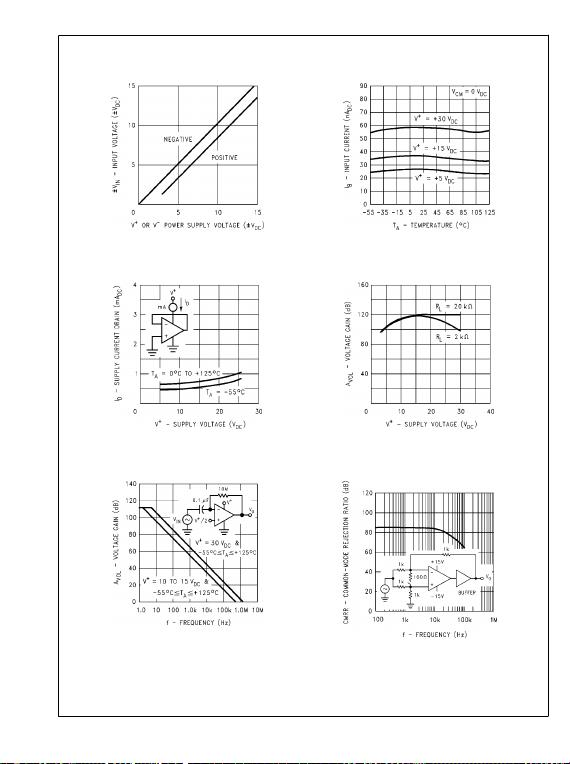Typical Performance Characteristics
Input Voltage Range
20150234
Input Current
20150235
Supply Current
20150236
Voltage Gain
20150237
Open Loop Frequency Response
20150238
Common-Mode Rejection Ratio
20150239
www.national.com 10
LM158QML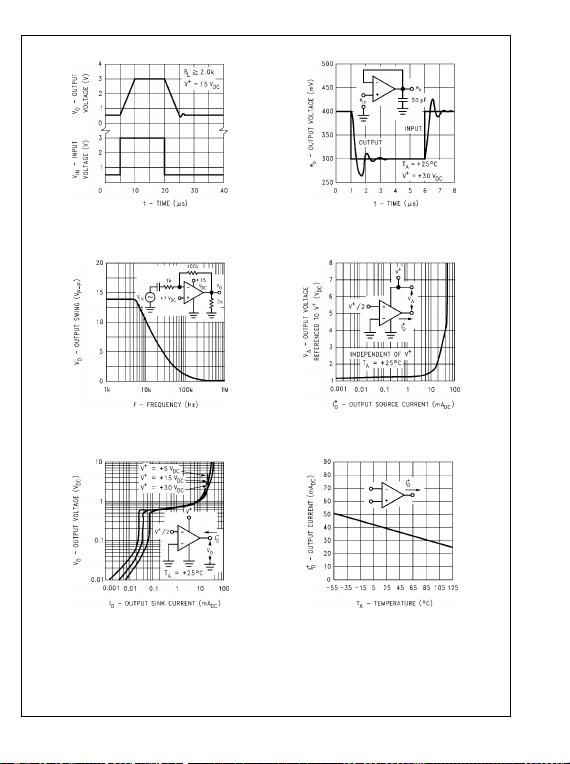Voltage Follower Pulse Response
20150240
Voltage Follower Pulse Response (Small Signal)
20150241
Large Signal Frequency Response
20150242
Output Characteristics Current Sourcing
20150243
Output Characteristics Current Sinking
20150244
Current Limiting
20150245
11 www.national.com
LM158QMLApplication Hints
The LM158 series are op amps which operate with only a
single power supply voltage, have true-differential inputs, and
remain in the linear mode with an input common-mode volt-
age of 0 VDC. These amplifiers operate over a wide range of
power supply voltage with little change in performance char-
acteristics. At 25°C amplifier operation is possible down to a
minimum supply voltage of 2.3 VDC.
Precautions should be taken to insure that the power supply
for the integrated circuit never becomes reversed in polarity
or that the unit is not inadvertently installed backwards in a
test socket as an unlimited current surge through the resulting
forward diode within the IC could cause fusing of the internal
conductors and result in a destroyed unit.
Large differential input voltages can be easily accommodated
and, as input differential voltage protection diodes are not
needed, no large input currents result from large differential
input voltages. The differential input voltage may be larger
than V+ without damaging the device. Protection should be
provided to prevent the input voltages from going negative
more than −0.3 VDC (at 25°C). An input clamp diode with a
resistor to the IC input terminal can be used.
To reduce the power supply current drain, the amplifiers have
a class A output stage for small signal levels which converts
to class B in a large signal mode. This allows the amplifiers
to both source and sink large output currents. Therefore both
NPN and PNP external current boost transistors can be used
to extend the power capability of the basic amplifiers. The
output voltage needs to raise approximately 1 diode drop
above ground to bias the on-chip vertical PNP transistor for
output current sinking applications.
For ac applications, where the load is capacitively coupled to
the output of the amplifier, a resistor should be used, from the
output of the amplifier to ground to increase the class A bias
current and prevent crossover distortion. Where the load is
directly coupled, as in dc applications, there is no crossover
distortion.
Capacitive loads which are applied directly to the output of the
amplifier reduce the loop stability margin. Values of 50 pF can
be accommodated using the worst-case non-inverting unity
gain connection. Large closed loop gains or resistive isolation
should be used if larger load capacitance must be driven by
the amplifier.
The bias network of the LM158 establishes a drain current
which is independent of the magnitude of the power supply
voltage over the range of 3 VDC to 30 VDC.
Output short circuits either to ground or to the positive power
supply should be of short time duration. Units can be de-
stroyed, not as a result of the short circuit current causing
metal fusing, but rather due to the large increase in IC chip
dissipation which will cause eventual failure due to excessive
junction temperatures. Putting direct short-circuits on more
than one amplifier at a time will increase the total IC power
dissipation to destructive levels, if not properly protected with
external dissipation limiting resistors in series with the output
leads of the amplifiers. The larger value of output source cur-
rent which is available at 25°C provides a larger output current
capability at elevated temperatures (see typical performance
characteristics) than a standard IC op amp.
The circuits presented in the section on typical applications
emphasize operation on only a single power supply voltage.
If complementary power supplies are available, all of the stan-
dard op amp circuits can be used. In general, introducing a
pseudo-ground (a bias voltage reference of V+/2) will allow
operation above and below this value in single power supply
systems. Many application circuits are shown which take ad-
vantage of the wide input common-mode voltage range which
includes ground. In most cases, input biasing is not required
and input voltages which range to ground can easily be ac-
commodated.
www.national.com 12
LM158QML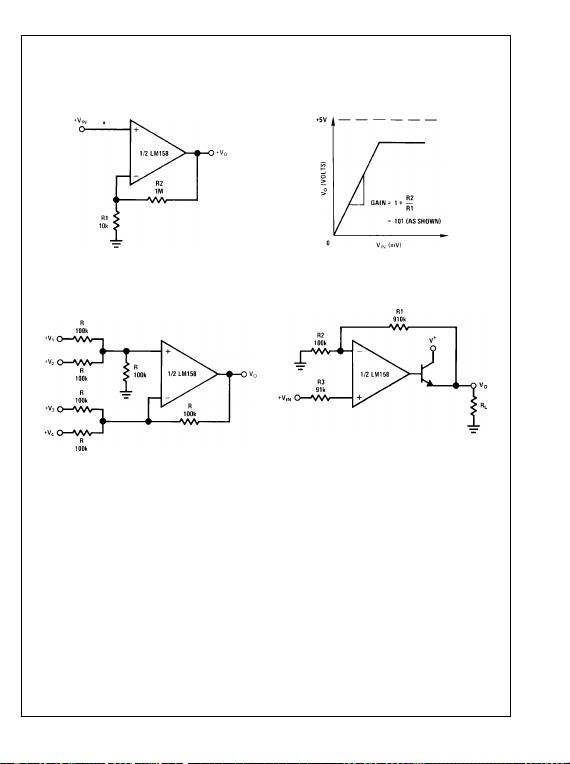Typical Single-Supply Applications
(V+ = 5.0 VDC)
Non-Inverting DC Gain (0V Output)
20150206
*R not needed due to temperature independent IIN
20150207
DC Summing Amplifier
(VIN'S 0 VDC and VO 0 VDC)
20150208
Where: VO = V1 + V2 −V3 − V4
(V1 + V2) (V3 + V4) to keep VO > 0 VDC
Power Amplifier
20150209
VO = 0 VDC for VIN = 0 VDC
AV = 10
13 www.national.com
LM158QML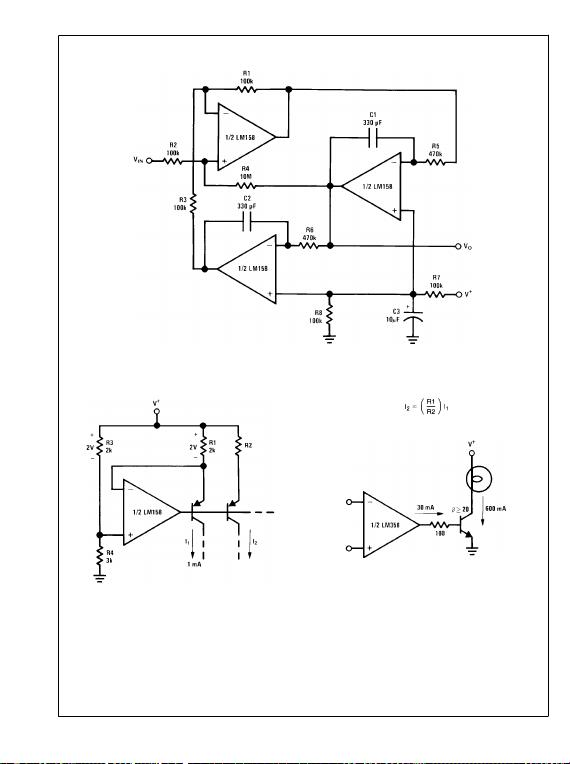BI-QUAD” RC Active Bandpass Filter
20150210
fo = 1 kHz
Q = 50
Av = 100 (40 dB)
Fixed Current Sources
20150211
Lamp Driver
20150212
www.national.com 14
LM158QML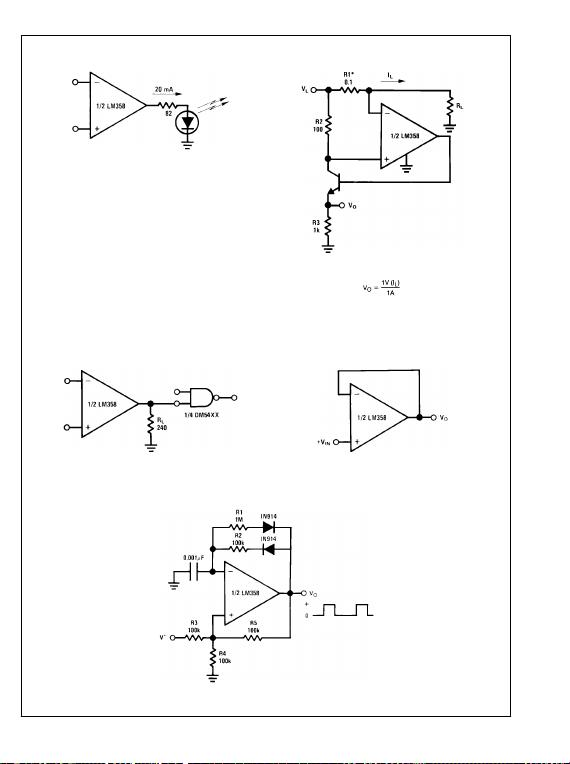LED Driver
20150213
Current Monitor
20150214
*(Increase R1 for IL small)
VL V+ −2V
Driving TTL
20150215
Voltage Follower
20150217
VO = VIN
Pulse Generator
20150216
15 www.national.com
LM158QML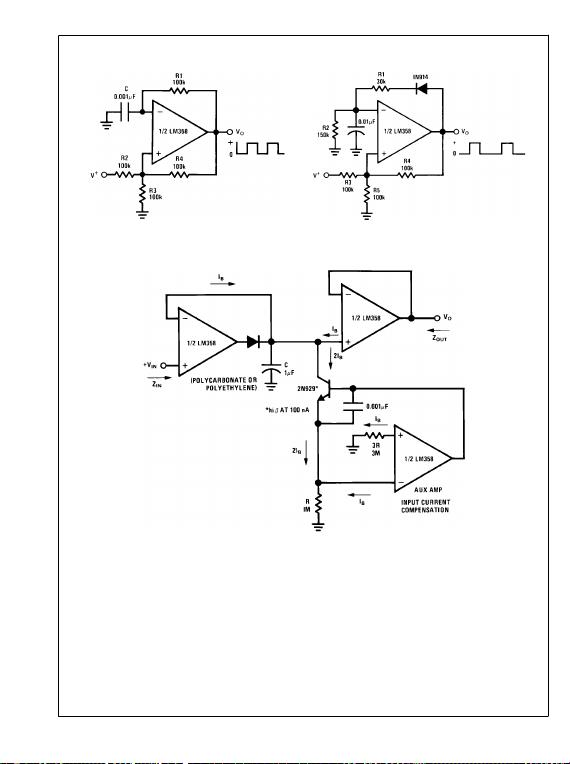Squarewave Oscillator
20150218
Pulse Generator
20150219
Low Drift Peak Detector
20150220
HIGH ZIN
LOW ZOUT
www.national.com 16
LM158QML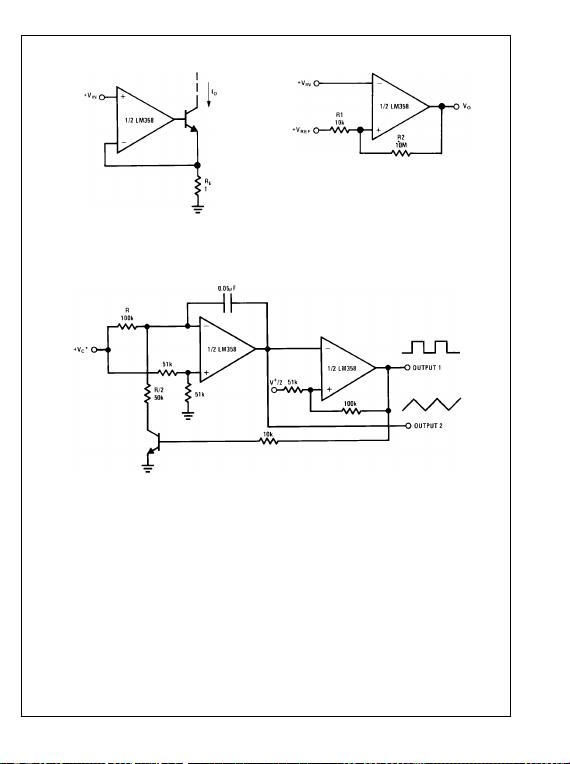High Compliance Current Sink
20150221
IO = 1 amp/volt VIN
(Increase RE for IO small)
Comparator with Hysteresis
20150222
Voltage Controlled Oscillator (VCO)
20150223
*WIDE CONTROL VOLTAGE RANGE: 0 VDC VC 2 (V+ −1.5V DC)
17 www.national.com
LM158QML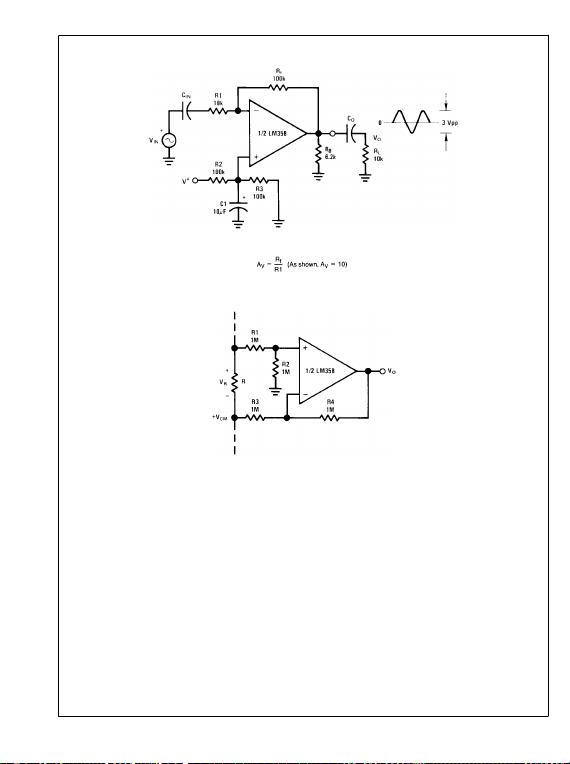AC Coupled Inverting Amplifier
20150224
Ground Referencing a Differential Input Signal
20150225
www.national.com 18
LM158QML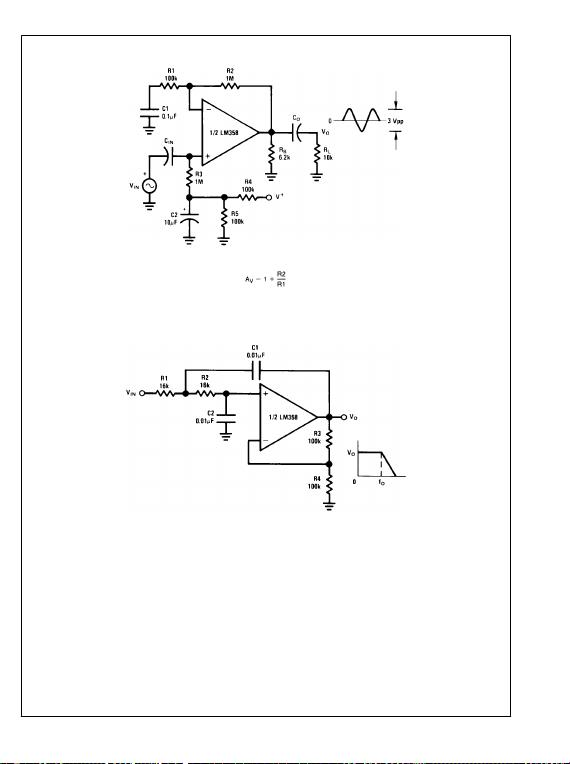AC Coupled Non-Inverting Amplifier
20150226
Av = 11 (As Shown)
DC Coupled Low-Pass RC Active Filter
20150227
fo = 1 kHz
Q = 1
AV = 2
19 www.national.com
LM158QML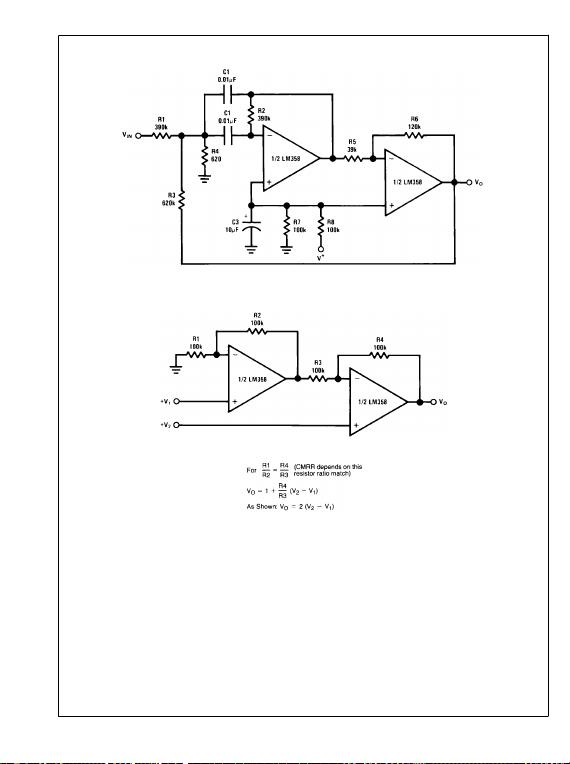Bandpass Active Filter
20150228
fo = 1 kHz
Q = 25
High Input Z, DC Differential Amplifier
20150229
www.national.com 20
LM158QML